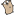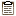## Go by Example: Interfaces

 Interfaces are named collections of method signatures.``package main`` ``````import ( "fmt" "math" )`````` Here’s a basic interface for geometric shapes. ``````type geometry interface { area() float64 perim() float64 }`````` For our example we’ll implement this interface on `rect` and `circle` types. ``````type rect struct { width, height float64 } type circle struct { radius float64 }`````` To implement an interface in Go, we just need to implement all the methods in the interface. Here we implement `geometry` on `rect`s. ``````func (r rect) area() float64 { return r.width * r.height } func (r rect) perim() float64 { return 2*r.width + 2*r.height }`````` The implementation for `circle`s. ``````func (c circle) area() float64 { return math.Pi * c.radius * c.radius } func (c circle) perim() float64 { return 2 * math.Pi * c.radius }`````` If a variable has an interface type, then we can call methods that are in the named interface. Here’s a generic `measure` function taking advantage of this to work on any `geometry`. ``````func measure(g geometry) { fmt.Println(g) fmt.Println(g.area()) fmt.Println(g.perim()) }`````` ``````func main() { r := rect{width: 3, height: 4} c := circle{radius: 5}`````` The `circle` and `rect` struct types both implement the `geometry` interface so we can use instances of these structs as arguments to `measure`. `````` measure(r) measure(c) }``````
 ``````\$ go run interfaces.go {3 4} 12 14 {5} 78.53981633974483 31.41592653589793`````` To learn more about Go’s interfaces, check out this great blog post.

Next example: Struct Embedding.